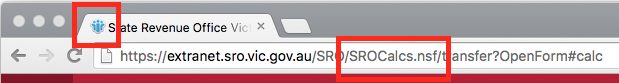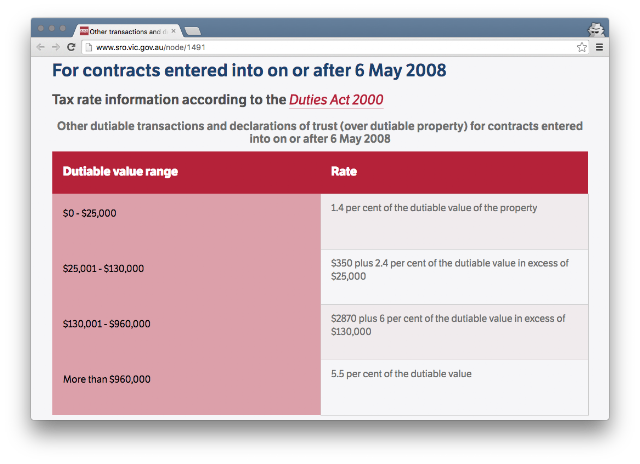Sunday, November 13, 2016

Excel formula for calculating stamp duty in Victoria, Australia (that'd be where Melbourne and good coffee is!)

I've been looking for a house to buy which means I don't have Saturday mornings anymore, and my laptop overheats because I have 97 Chrome tabs all open at domain.com.au and realestate.com.au. (one for every affordable property remaining in Australia). As a Sydneysider, I was shocked by the low cost of housing in Victoria. A house for less than \$1 million?! Surely a misprint!

Long story short, the Excel formula for calculating stamp duty in the state of Victoria is:

It's based on the duty ranges published by the Victoria State Revenue Office. The SRO also have a duty calculator which appears to be a Lotus Notes application. Go figure. The formula assumes you are an Australian citizen and are not eligible for any sort of first home owner's benefit.BWAHAHAHAH LOTUS NOTES APPNO SERIOUSLY WHAT CENTURY ARE WE IN

Here are the components of the Excel formula.

A1: This is the cell that contains the property value or purchase price (whichever is higher, otherwise people would sell their properties on paper for the price of a Big Mac.)

ROUND: Land duty operates on percentages, and the tax office don't bother with cents.

IF(IF(IF(x))): Stamp duty operates on a sliding scale. There are four valuable duty ranges, therefore we need 3 IF() statements to determine which range the property has liabilities in. Why not four? The first IF() evalutes whether the property is range 4 or 3, the second IF() evaluates whether the property is in range 3 or 2, and the final IF() evaluates whether the property is in range 2 or 1.

The 960000, 130001 and 25001 magic numbers: These are the dutiable value ranges.

• If the property is below \$25,000, you're subject to range 1.
• If the property is above \$25,000 but below \$130,001, it's subject to range 1 and 2.
• If the property is above \$130,001 but below \$960,001, it's subject to ranges 1 and 2 and 3.
• If the property is above \$960,001, it's subject to range 4 only.Wasn't transfer duty supposed to be abolished with GST?!

The 0.055, 0.06, 0.024 and 0.014 magic numbers: These are the duty amounts.

• 0.055 is 5.5%
• 0.06 is 6.0%
• 0.024 is 2.4%
• 0.014 is 1.4%

0.06*(A1-130000), 0.025*(A1-25000): When calculating the stamp duty liability for a range, you don't calculate the liability based on the entire property value; you only calculate it on the liability within that range. Example:
• The property price is \$26,000. Liability for the first \$25,000 is 1.4%. For the \$1000 over this amount, the liability is calculated 2.4%. To calculate the 2.4% liability, you'd subtract \$25,000 from the total property value.

The 350 and 2870 magic numbers: This is the residual amount from the previous range.

• If you pay \$25,001, your property value is in range 2, and the total liability from range 1 is \$350. Your range 2 liability is calculated on top of that.
• If you pay \$130,000, your property value is in range 3, and the total liability from range 1 and 2 is \$2870. Your range 3 liability is calculated on top of that.
• It would be cleaner if I updated this formula so that the residual amount was calculated live. I guess I have a reason to update this blog post now. UPDATED: below!

Although I have tested my Excel formula against a number of values, you should compare its output against the official SRO website calculator before making any decisions with a financial impact larger than a McDonalds meal.

UPDATE: Yes, you can replace the 350+ and 2870+ components of the formula. No, it's definitely not cleaner. It makes the formula more complex and unmaintainable, which is what you don't want in Excel because the Excel formula bar has all the readability of hieroglyphics.

Here's what it looks like long form.

Why is 350+ replaced with (0.014*25000)? Assuming the property costs more than \$25,000, the maximum liability of range 1 is \$350 is 1.4% of a \$25,000. This can be represented in Excel as plain jane 350, or it can be expressed long form.

Why is 2870+ replaced with (0.014*25000)+(0.024*(130000-25000))? Assuming the property costs more than \$130,000, the total liability of range 1 and 2 is \$2870. \$2870 is made up of \$350 (maximum liability of range 1) and \$2520 (maximum liability in range 2).

1.Thank you very much.

2.this is fantastic - thanks mate!

3.Thank you for the detailed explanation. Much appreciated.

4.excellent, has saved me an evening of hell, thanks